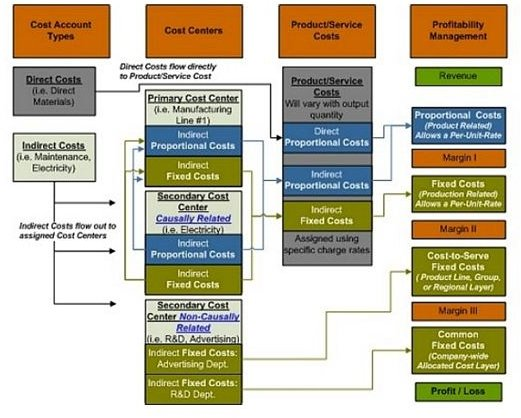# How to Calculate FIFO Inventory Costs When Determining the Year-End Balance of the Inventory Account

## What is FIFO?The FIFO inventory valuation method, or first-in first-out, is one of a number of inventory costing methods. It is used to facilitate the valuation of merchandise at the end of the year in the Inventory account. When determining how to calculate FIFO inventory costs, the basic assumption is that the first units of merchandise added to the physical inventory are going to be the first units removed during the course of business. The balance sheet Inventory account at the end of the year would then reflect the most recent costs matched to the most recent units produced, or purchased and placed in stock.

## Ending Inventory Valuation Using FIFO

Two inventory systems can be used for the FIFO inventory valuation method, and other inventory costing methods. They are the periodic inventory system, and the perpetual inventory system. The periodic inventory system does not update the Inventory account regularly, but instead uses a purchases account to record purchases of merchandise that are added to the physical inventory. The purchases account is closed at the end of the year, and the Inventory account is then updated. A Cost of Goods Sold account isn’t necessary for this system so there is no way of telling what the Inventory account amounts are during the course of the year.

## The Perpetual Inventory System

The perpetual inventory system, unlike the periodic inventory system, continuously records changes to the Inventory account. The account is increased by purchases of merchandise from suppliers, and reduced by sales of merchandise to customers.

The purchases account isn’t necessary in this system, but there is the Cost of Goods Sold account that is debited when merchandise is removed from the inventory and sold. This actually consists of two journal entries:

• The recording of the sale to the Sales account (a credit), and to the Cash, or Accounts Receivable accounts (a debit).
• The reduction of the Inventory account (a credit), and an addition to the Cost of Goods Sold account (a debit).

## The Periodic Inventory System

Calculating the ending inventory costs using periodic FIFO is fairly straightforward. The following example shows this.

100,000 units beginning inventory @ \$5.00 ea. = \$ 500,000

200,000 units purchased @ \$5.50 ea. = \$1,100,000

(50,000) units sold @ ? = ?

100,000 units purchased @ \$5.75 ea. = \$ 575,000

(75,000) units sold@ ? = ?

## Calculating Units in Ending inventory

The number of units in the ending inventory would be:

Ending Inventory = Beginning Inventory + Units Purchased – Units Sold

275,000 units = 100,000 units + 300,000 units – (50,000 units + 75,000 units)

## Determine the Cost of Each Sale

To determine the costs of each sale in this example it helps to consider purchases of inventory as forming three tiers:

Tier 1: 100,000 units (beginning inventory)

Tier 2: 200,000 units (first purchase)

Tier 3: 100,000 units (second purchase)

## First Sale of Goods in Inventory

The cost of the 50,000 units that were first sold would be determined by assigning the earliest purchase price to them. This would happen to be \$5.00 per unit from the first tier, the beginning inventory. The cost of the sale would be \$250,000:

50,000 units x \$5.00 = \$250,000

The the remaining balance of the beginning Inventory would be 50,000 units:

100,000 units – 50,000 units = 50,000 units.

## Second Sale of Goods in Inventory

The cost of the 75,000 units sale involves two tiers. The first 50,000 units would be costed at \$5.00 per unit from the remainder of the first tier (beginning inventory), and the next 25,000 units would be costed from the second tier (200,000) of inventory at \$5.50. The calculation would be:

Remainder of tier 1: 50,000 units @ \$5.00 = \$250,000

From tier 2: 25,000 units @ \$5.50 = \$137,500

The total sale would be: \$387,500

## Ending Inventory Balance

The value of the ending inventory would then be \$1,787,000:

\$1,787,000 = \$500,000 + \$1,675,000 – (\$250,000 + 137,500)

## Summary

The cost flow using FIFO assigns the oldest purchase prices to inventory merchandise when they are sold. How to calculate FIFO inventory costs is dependent upon the existing inventory system being used.

The periodic inventory system uses a Purchases account to record purchases from suppliers, and adjusts the Inventory account for additions, or reductions at the end of the year. The perpetual inventory system continually updates the Inventory account throughout the year debiting a Cost of Goods Sold account and crediting the Inventory account when merchandise is sold. The systems are different, but when calculating inventory costs using periodic FIFO, or perpetual FIFO shows no differences in the Inventory account on the balance sheet.

## Citations

"Inventory Chapter 8," PrinciplesofAccounting.com. Retrieved August 2, 2010 from the World Wide Web: https://www.principlesofaccounting.com/chapter%208.htm#inventory%20costing%20methods

"Comparison of Inventory Valuation Methods," Accounting Study Guide Online, 2010. Accounting Courses Online. Retrieved August 2, 2010 from the World Wide Web: https://accountinginfo.com/study/inventory/inventory-120.htm

"Inventory and Cost of Goods Sold," AccountingCoach.com, 2010. Retrieved August 2,2010 from the World Wide Web: https://www.accountingcoach.com/online-accounting-course/12Xpg02.html#periodic-fifo